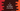# How to get the length of an array in C# Length of an array in C:

In C, we need the length of an array to iterate over the elements. Normally, this length is predefined or we take the length as an input from the user. But how can we get the length of an array of unknown size ? Let’s check that !

## sizeof :

The sizeof operator is used to compute the size of a value that we can provide. For example, you can get the size of an integer, character or an array.

You must be aware of that the memory allocated for a data type is different in different architectures. For example, if the size of an integer is 4 bytes on my laptop, it might different on your laptop. So, the result of sizeof may vary.

Let’s consider the below C program :

``````#include <stdio.h>
int main()
{
int i = 10;
int arr = {1,4,5};
printf(“Size of i is = %d\n”, sizeof(i));
printf(“Size of arr is = %d\n”, sizeof(arr));
return 0;
}``````

Here, we have one integer i and one integer array arr. We are printing the value of sizeof for both of these values. For my laptop, it produces the below output :

``````Size of i is = 4
Size of arr is = 12``````

So, in my machine, the size of an integer number is 4 bytes. The array contains three integers and its size is three times 4 i.e. 12.

Now, if we want to find out the length of an array, we can simply calculate the sizeof array and divide that value by the sizeof any of its item. That will give us the result.

``````#include <stdio.h>
int main()
{
int arr1 = {1,4,5};
int arr2 = {1,4,5};
printf(“Length of arr1 is = %d\n”, sizeof(arr1)/sizeof(arr1));
printf(“Length of arr2 is = %d\n”, sizeof(arr2)/sizeof(arr2));
return 0;
}``````

It will print :

``````Length of arr1 is = 3
Length of arr2 is = 5``````

### Avoid using this method with functions :

If we are passing one array as parameter to a different function, it will give us different result. Because, if we pass one array to a function, it is considered as a pointer. For the below example :

``````#include <stdio.h>
void findSize(int arr[]){
printf(“%d\n”, sizeof(arr));
}
int main()
{
int arr1 = {1,4,5};
findSize(arr1);
return 0;
}``````

It will print the size of a pointer. It prints 8 on my laptop.

If you are using functions as like the above, always pass the size of the array along with it.